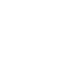# Apr 16 SAT Question of the Day and ACT QotD

If you are reading this in an email you received from me, do not click the link to sat.collegeboard.org below. Use the link to my website that is farther down on the email. If you are seeing this in my blog, do the SAT Question of the Day by clicking on this link:

http://sat.collegeboard.org/practice/sat-question-of-the-day?src=R&questionId=20130416 (This link takes you to today’s question. If you use my archive, you will see the question related to my SAT explanation for that date.)

The answer is D.  If you recognize the function as a downward-facing parabola and understand the characteristics of such a graph, you can do it pretty easily the “math teacher” way.  The SAT folks explain it well; so, take a look at their explanation and that will save me the time of doing some typing.  BUT…

Many (or perhaps most — based on the fact that only 35% of students are getting this question correct) test takers won’t see how to do it that way!  Use the Wizard’s strategy (Pillar V) that takes advantage of the answer being on the page.  Whenever the question asks something like, “what is the value of…,” you just check out the answers.  Plug them into the function (or equation) in this case.

Most importantly, don’t just guess 200 since that is the largest answer.  Remember since there is a squared value on the right side of the function/equation, it is not a straight line.  When you plug in 10, 20, 50, and 100, the answer keeps getting larger but when you plug in 200, you get 0.  Just be careful.

On a practical level, I would fire this manager!  Who would ever think you would want a product that would start costing the company money after selling a certain number of the items?  On the other hand, maybe I should fire the test question writer.  This is the only time that I can recall that the situation in the question didn’t make sense.  The questions are supposed to reflect reality.

Let’s go visit the ACT site and see what they’ve cooked up for us today.

http://www.act.org/qotd/ (The ACT staff does not put a date on their questions so if you click on an archived blog, you’ll get today’s question and the old explanation. Sorry. The SAT staff has dated their questions; so, the archive is helpful. The ACT folks simply don’t do that.)

Oops, no calculator is needed, just some thinking.

The answer is H.  Since the rate formula is D=rt, this is a pretty simple question if you remember how to multiply exponents.  They give you the rate and time and ask you to calculate distance.  4 times 1 is 4 and adding the exponents you get 8-6=2.  That’s answer H.

Maybe you forgot the formula.  What do you do now?  Quickly think about what you would have done with easy numbers.  If the question had asked if you were driving your car 30 miles per hour for two hours, how far would you go, I bet you would easily multiply 30 times 2 in your head and get 60.  Well, there’s the formula!  You knew to multiply rate times time for this simple question.  Now just apply the same math to the ACT question since they give you rate and time.  Don’t be intimidated (Pillar VI) by their confusing numbers for rate and time.  The math calculation will be done the same way.  Use the Wizard’s strategy of reducing their complicated situation to an everyday situation using small numbers to see how you would do it.  Then apply your calculation process to their question.  You’ll be pleased with how that technique is going to raise your score.